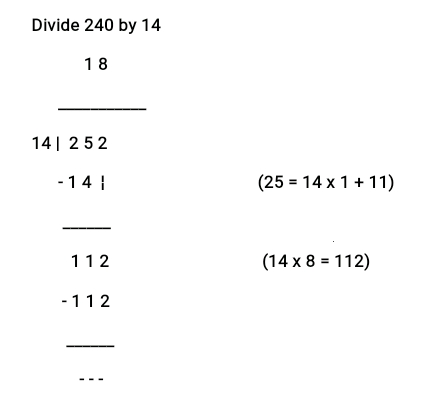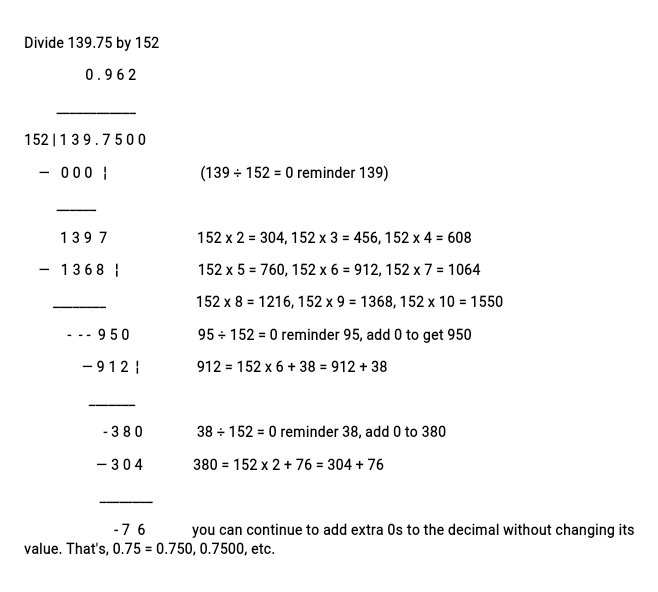# Division of Whole Numbers by 2-digit and 3-digit Numbers

FIRST TERM

TENTH WEEK

BASIC 6

THEME: BASIC OPERATIONS

TOPIC: Division of Whole Numbers and Decimals

Lead pupils to master how to use the long division to divide 4 or 5 digit numbers by 2-digit whole numbers. Explain each step taken and guide them through the examples.

[bibblio style=”bib–default bib–hover bib–white-label bib–font-arial bib–size-18 bib–grd-6″ query_string_params=”e30=” recommendation_type=”optimised”]

PERFORMANCE OBJECTIVES

• By the end of the lesson, the pupils should have attained the following objectives (cognitive, affective and psychomotor) and be able to divide whole number and decimal by 2-digit and 3-digit numbers.

ENTRY BEHAVIOR

The pupils are required to already have learned multiplication.

INSTRUCTIONAL MATERIALS

The teacher will teach the lesson with the aid of flash cards and charts of multiplication table.METHOD OF TEACHING

1. Teacher’s – Pupil’s Activities
2. Pupil’s – Teacher’s Activities

REFERENCE MATERIALS

1. Scheme of Work
2. 9 – Years Basic Education Curriculum
3. New Method Mathematics Book 6
4. All Relevant Material
5. Online Information

CONTENT OF THE LESSON

Lead pupils to master how to use the long division to divide 4 or 5 digit numbers by 2-digit whole numbers. Explain each step taken and guide them through the examples.

LESSON ONE

DIVISION OF WHOLE NUMBERS BY 2-DIGIT NUMBER

Example 1Therefore,

185 divided by 15 = 12 Reminder 5

[bibblio style=”bib–default bib–hover bib–white-label bib–font-arial bib–size-18 bib–grd-6″ query_string_params=”e30=” recommendation_type=”optimised”]

Example 2Exercises

Divide:

1. 252 by 18
2. 252 by 16

LESSON TWO

DIVISION OF WHOLE NUMBERS BY 3-DIGIT NUMBER

ExampleExercises

Divide:

1. 2340 by 156
2. 2340 by 150

LESSON THREE

DIVISION OF DECIMAL BY 2-DIGIT AND 3-digit WHOLE NUMBERS

Thing you need to know:

1. Divide decimals as with whole numbers,
2. Place the decimal point in the answer directly above the decimal point in the dividend.

Example 1Example 2Exercises

Divide:

1. 72.38 by 47
2. 72.38 by 128

LESSON FOUR

Further Practice, as stated in the recommended books.

[bibblio style=”bib–default bib–hover bib–white-label bib–font-arial bib–size-18 bib–grd-6″ query_string_params=”e30=” recommendation_type=”optimised”]

PRESENTATION

• To deliver the lesson, the teacher adopts the following steps:
1. To introduce the lesson, the teacher revises the previous lesson. Based on this, he/she asks the pupils some questions;
2. Guides the pupils to divide whole number by 2-digit numbers;
3. Pupil’s Activities – Divide whole number by 2-digit numbers.
4. Guides the pupils to solve problems on division of whole numbers and decimals by 2-digit and 3-digit numbers;
5. Pupil’s Activities – Solve problems on division of whole numbers and decimals by 2-digit and 3-digit numbers.
6. Guides the pupils to solve quantitative problems on division of whole numbers and decimals by 2-digit and 3-digit numbers;
7. Pupil’s Activities – Solve quantitative problems on division of whole numbers and decimals by 2-digit and 3-digit numbers.

CONCLUSION

• To conclude the lesson for the week, the teacher revises the entire lesson and links it to the following week’s lesson – revision.

LESSON EVALUATION

Pupils to:

1. solve given problems on division by 2-digit and 3-digit number;
2. solve problem on quantitative aptitude involving division.